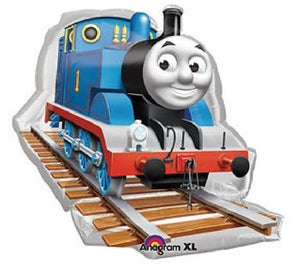# Loonballoon Thomas The Tank Train Engine Track 36 Large Figure Mylar Foil Party Balloon

Vendor
LoonBalloon
Regular price
Sold out
Sale price
\$22.62
Quantity must be 1 or more

• L

• o

• o

• n

• B

• a

• l

• l

• o

• o

• n

• T

• H

• O

• M

• A

• S

• t

• h

• e

• T

• a

• n

• k

• T

• R

• A

• I

• N

• E

• n

• g

• i

• n

• e

• T

• r

• a

• c

• k

• 3

• 6

• L

• a

• r

• g

• e

• F

• i

• g

• u

• r

• e

• M

• Y

• L

• A

• R

• F

• o

• i

• l

• P

• a

• r

• t

• y

• B

• A

• L

• L

• O

• O

• N

LoonBalloon THOMAS the Tank TRAIN Engine Track 36 Large Figure MYLAR Foil Party BALLOONGreat for parties, birthdays, holidays, and special occasions. Mix and match with latex and foil balloons to create fun, festive balloon bouquets for easy party decorations and fun!